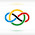### 1975 IMO SL #2

We consider two sequences of real numbers $x_{1} \geq x_{2} \geq \ldots \geq x_{n}$ and $\ y_{1} \geq y_{2} \geq \ldots \geq y_{n}.$ Let $z_{1}, z_{2}, .\ldots, z_{n}$ be a permutation of the numbers $y_{1}, y_{2}, \ldots, y_{n}.$ Prove that $\sum \limits_{i=1}^{n} ( x_{i} -\ y_{i} )^{2} \leq \sum \limits_{i=1}^{n}$ $( x_{i} - z_{i})^{2}.$

Since there are only finitely many arrangements of the $z_i$'s, suppose that $z_1, \cdots, z_n$ is the permutation for which $$\sum_{i=1}^n (x_i-z_i)^2$$ is minimal. We claim that in this case, $i<j$ implies $z_i \ge z_j$, from which the desired statement directly follows.

For the sake of contradiction, assume that this is not true. That is, $i<j$ does not necessarily imply $z_i \ge z_j$ in this case, or that it implies $z_i<z_j$. Then we have $$(x_i-z_j)^2+(x_j-z_i)^2=(x_i-z_i)^2+(x_j-z_j)^2+2(x_iz_i+x_jz_j-x_iz_j-x_jz_i)$$ $$=(x_i-z_i)^2+(x_j-z_j)^2+2(x_i-x_j)(z_i-z_j)$$ $$\le (x_i-z_i)^2+(x_j-z_j)^2,$$ which is clearly a contradiction.

So, have proved that $i<j \implies z_i \ge z_j$, and hence we are done. $\square$

1.Anonymous3/17/2022

very clean

btw u should stream on twitch sometimes
it would be easier to explain
because of course on camera it is much easier to explain as well as understand

2.@anonymous

you're right.

i think i will stream starting this saturday.
but on twitch i will do problems that are not from the IMO, because those I have explained in this blog.